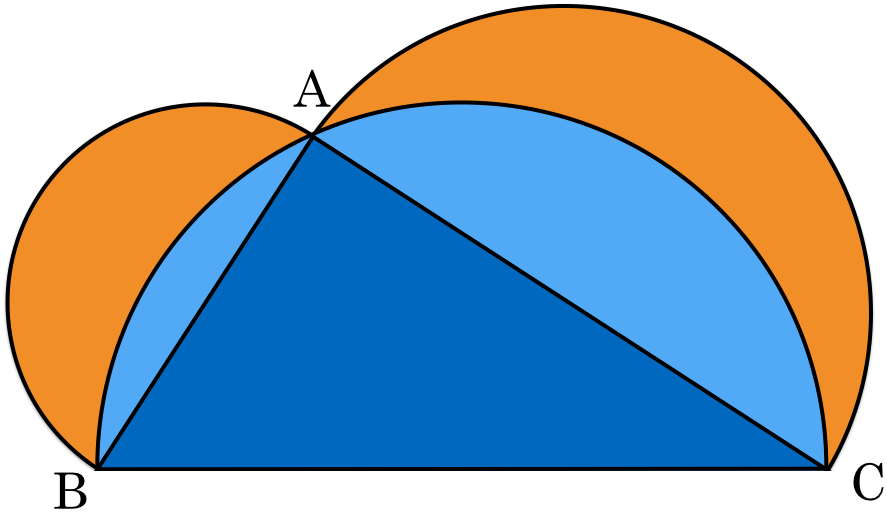# The two moons

Geometry Level 2Consider a triangle $ABC$ with right angle $A$, $BC=5$ and $AC=4.$ Three semicircles are drawn as in the figure above. Find the sum of the areas of the orange shaded regions.

×